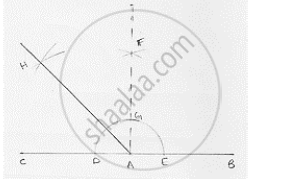# Using Ruler and Compasses Only, Draw an Angle of Measure 135°. - Mathematics

Using ruler and compasses only, draw an angle of measure 135°.

#### SolutionSteps of construction:
1. Draw a line segment AB and produce BA to point C.
2. With center A and any radius draw arc which intersect AC at D and AB at E.

3. With center D and E and radius more than 1/2DE draw two arcs which intersect each other at F.
4. Join FA which intersect the arc in (2) at G.
5. With centers G and D and radius more than 1/2GD draw two arcs which intersect each other at H.
6. Join HA

∴ ∠ HAB = 135°

Concept: Basic Constructions
Is there an error in this question or solution?

#### APPEARS IN

RD Sharma Mathematics for Class 9
Chapter 16 Constructions
Exercise 16.2 | Q 8 | Page 9

Share